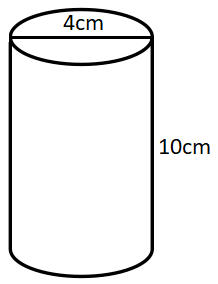# Surface Area

The surface area of a 3D shape is the sum of the areas of each face. Sureace area is also measured in square units such as square centimeters ( $$cm^2$$ ), square inches ( $$in^2$$ ) etc..

## Surface Area of Common Shapes

Let's look at how to calculate the surface area of the shapes below:

### Cube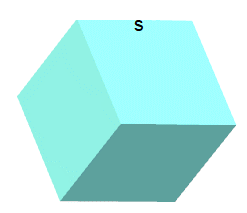The surface area of a cube with length s is calculated by:

$$SA = 6 s^2$$

### Cuboid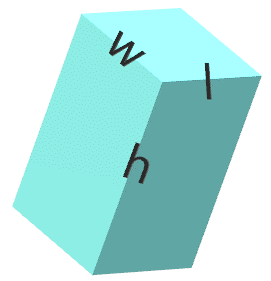The surface area of a cuboid (rectangular prism) with length l, width w and height h is calculated by:

$$SA = 2 (l w + l h + w h)$$

### Cone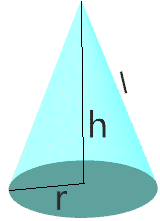The surface area of a cone with height h, slant l and radius r is calculated by:

$$SA = \pi r (l + r)$$

### Cylinder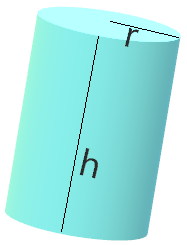The surface area of a cylinder with radius r and height h is calculated by:

$$SA = 2 \pi r (h + r)$$

### Triangular Prism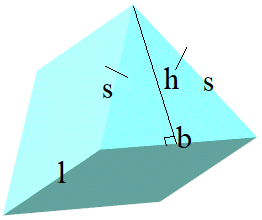The surface area of a triangular prism with two equal sides s, base b, height h and length l is calculated by:

$$SA = bh + 2ls + lb$$

### Sphere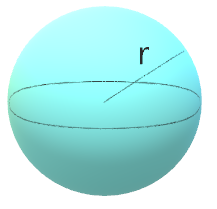The surface area of a sphere with radius r is calculated by:

$$SA = 4 \pi r^2$$

## Surface Area Using Nets

The net of an 3D shape is formed when the shape is unfolded along its edges and its faces are laid out in a pattern in 2D. Nets are helpful to visualize the different faces of a 3D object to calculate the surface area.

Let's draw a net for the shape below.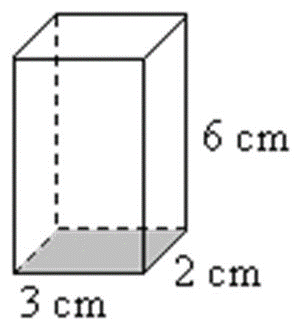We have to unfold the 3D object to get the net shape below: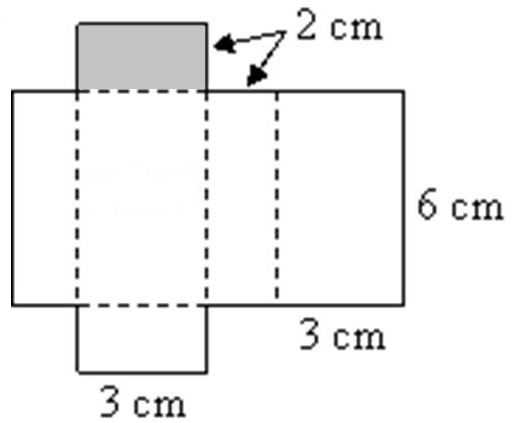You can see that the original 3D shape is made up of 6 rectangles. We can calculate the area of each using $$A = lw$$. Lastly, we add them all up to get the surface area.

$$SA = (2)(6) + (3)(6) + (2)(6) + (3)(6) + (3)(2) + (3)(2)$$

$$SA = 2 ( (2)(6) + (3)(6) + (3)(2) )$$

$$SA = 2 (12 + 18 + 6)$$

$$SA = 72 \; [\text{cm}^2]$$

The surface area is $$72 \; [\text{cm}^2]$$ .

A sphere has a radius $$6$$ inches, what is its surface area?

Juan is making a yard sign in the shape of a rectangular prism. The yard sign will be $$60\;[cm]$$ long, $$40\;[cm]$$ high and $$5 \;[cm]$$ thick. The cost of painting the yard sign is \$0.002 per square cm. How much does Juan have to spend to paint the yard sign?

Draw a net for the shape below and calculate the surface area.